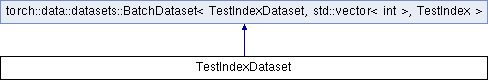Caffe2 - C++ API A deep learning, cross platform ML framework
TestIndexDataset Struct Reference
Inheritance diagram for TestIndexDataset:## Public Member Functions

TestIndexDataset (size_t size)

std::vector< int > get_batch (TestIndex index) override
Returns a batch of data given an index.

torch::optional< size_t > size () const override
Returns the size of the dataset, or an empty optional if it is unsized.Public Member Functions inherited from torch::data::datasets::BatchDataset< TestIndexDataset, std::vector< int >, TestIndex >
MapDataset< TestIndexDataset, TransformType > map (TransformType transform)&
Creates a `MapDataset` that applies the given `transform` to this dataset.

MapDataset< TestIndexDataset, TransformType > map (TransformType transform)&&
Creates a `MapDataset` that applies the given `transform` to this dataset.

## Data Fields

std::vector< int > dataPublic Types inherited from torch::data::datasets::BatchDataset< TestIndexDataset, std::vector< int >, TestIndex >
using SelfType = TestIndexDataset

using BatchType = std::vector< int >

using BatchRequestType = TestIndexStatic Public Attributes inherited from torch::data::datasets::BatchDataset< TestIndexDataset, std::vector< int >, TestIndex >
static constexpr bool is_stateful

## Detailed Description

Definition at line 784 of file dataloader.cpp.

The documentation for this struct was generated from the following file: